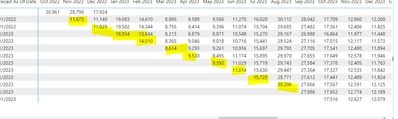cancel
Showing results for
Did you mean:Frequent Visitor

## Dax Conditional Formatting to Highlight Last ValueThis is an illustration of a forecast. Forecast as of date which comes from my historical forecast table represents the date in which a forecast was updated(In this case the forecast is updated every month) and the matrix displays those values in columns by forecasted month using my Date table. I would like to be able to highlight the last value updated from that month but I can not wrap my head around the logic to apply in a dax formula.

1 ACCEPTED SOLUTIONSuper User
``````Measure Conditional Format =
VAR __ForecastMonth = MAX('Historical Forecast'[Forecast As Of Date])
VAR __Date = MAX( 'Date'[Date] )
VAR __MaxDate = MAXX ( FILTER( ALL( 'Date' ), 'Historical Forecast'[Forecast as of Date] = __ForecastMonth ), [Date] )
VAR __Result = IF( __Date = __MaxDate, 1, 0 )
RETURN
__Result``````

Become an expert!: Enterprise DNA
External Tools: MSHGQM
Latest book!:
Mastering Power BI 2nd EditionDAX is easy, CALCULATE makes DAX hard...
3 REPLIES 3Frequent Visitor

This is what I compliled however, I received this error message

Measure Conditional Format =
VAR __ForecastMonth = MAX('Historical Forecast'[Forecast As Of Date])
VAR __Date = MAX( 'Date'[Date] )
VAR __MaxDate = MAXX ( FILTER( ALL( 'Date' ), 'Historical Forecast'[Forecast as of Date] = __ForecastMonth ), [Date] )
VAR __Result = IF( __Date = __MaxDate, 1, 0 )
RETURN
MAXX('Historical Forecast', __Result)

A single value for column 'Forecast as of Date' in table 'Historical Forecast' cannot be determined. This can happen when a measure formula refers to a column that contains many values without specifying an aggregation such as min, max, count, or sum to a single unitSuper User
``````Measure Conditional Format =
VAR __ForecastMonth = MAX('Historical Forecast'[Forecast As Of Date])
VAR __Date = MAX( 'Date'[Date] )
VAR __MaxDate = MAXX ( FILTER( ALL( 'Date' ), 'Historical Forecast'[Forecast as of Date] = __ForecastMonth ), [Date] )
VAR __Result = IF( __Date = __MaxDate, 1, 0 )
RETURN
__Result``````

Become an expert!: Enterprise DNA
External Tools: MSHGQM
Latest book!:
Mastering Power BI 2nd EditionDAX is easy, CALCULATE makes DAX hard...Super User

@JanaeWalker864 Tough to be exact since I have no idea about your table and column names. But maybe something like:

``````Measure Conditional Format =
VAR __ForecastMonth = MAX('Forecast'[MonthYear])
VAR __Date = MAX( 'Dates'[Date] )
VAR __MaxDate = MAXX ( FILTER( ALL( 'Dates' ), [MonthYear] = __ForecastMonth ), [Date] )
VAR __Result = IF( __Date = __MaxDate, 1, 0 )
RETURN
__Result``````

You can then create a conditional formatting rule based on this measure where 1 is the last date in each forecast month.

Become an expert!: Enterprise DNA
External Tools: MSHGQM
Latest book!:
Mastering Power BI 2nd EditionDAX is easy, CALCULATE makes DAX hard...Announcements#### Exclusive opportunity for Women!

Join us for a free, hands-on Microsoft workshop led by women trainers for women where you will learn how to build a Dashboard in a Day!#### Power Platform Conference-Power BI and Fabric Sessions

Join us Oct 1 - 6 in Las Vegas for the Microsoft Power Platform Conference.Top Solution Authors
Top Kudoed Authors
Users online (1,928)